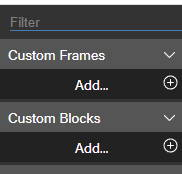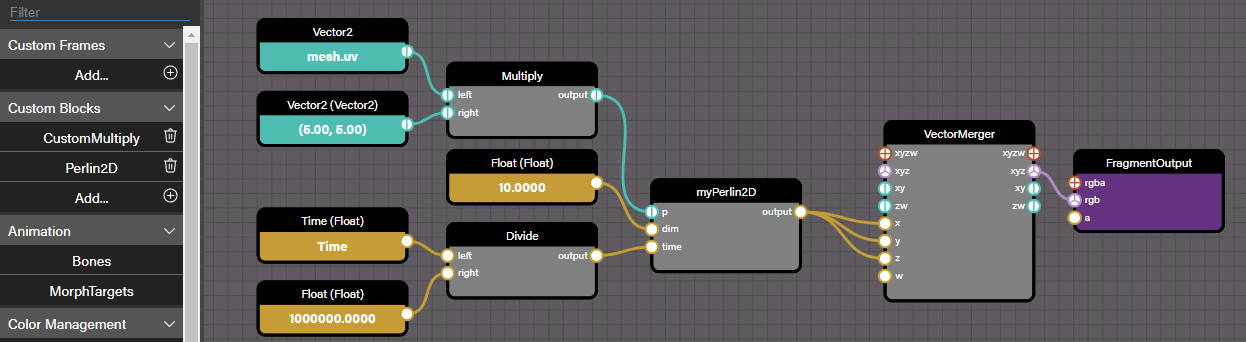# Node Material Editor Custom Blocks

## Custom Blocks

Starting with v5.0 you can now create custom blocks by wrapping some plain GLSL code. It can come in handy when you have some complicated code that could be difficult to recreate with the existing node material blocks.

### Register Custom Blocks with the NME

At the time, a custom block is described by a .json file that you must provide when registering a new custom block in the node material editor:When clicking on the "+" icon to add (register) a new custom block, you will be requested for the .json file that describes the block.

Here's how a Multiply custom block could be implemented:

{    "name": "CustomMultiply",    "comments": "Multiplies the left and right inputs of the same type together",    "target": "Neutral",    "inParameters": [        {            "name": "left",            "type": "AutoDetect"        },        {            "name": "right",            "type": "AutoDetect"        }    ],    "outParameters": [        {            "name": "output",            "type": "BasedOnInput",            "typeFromInput": "left"        }    ],    "inLinkedConnectionTypes" : [        {            "input1": "left",            "input2": "right",            "looseCoupling": false        }    ],    "functionName": "multiply_{TYPE_left}",    "code": [        "{TYPE_output} myHelper_{TYPE_left}({TYPE_left} l, {TYPE_right} r) { return l * r; }",        "void multiply_{TYPE_left}({TYPE_left} l, {TYPE_right} r, out {TYPE_output} result) {",        "   result = myHelper_{TYPE_left}(l, r);",        "}"    ]    }

Most of the properties should be self-explanatory. Here are the possible values for some of the properties:

• target: Neutral, Vertex, Fragment, VertexAndFragment
• in/out parameters: Float, Int, Vector2, Vector3, Vector4, Color3, Color4, Matrix, Object, AutoDetect, BasedOnInput
• in parameters only: sampler2D, samplerCube

As this block allows any type of input (Float, Vector3, etc as the AutoDetect value shows for the left and right parameter types), the type of output is set to BasedOnInput to inherit the value of the input that will be plugged to left (value of typeFromInput) at runtime. Also, we want the types of the left and right inputs to be the same (once an input is plugged, the other one should inherit the same type), that's why we have a inLinkedConnectionTypes section in the file.

As the types are not known at the time we create the .json file, we must reference them in the GLSL code by using the special syntax {TYPE_XXX} where XXX is the name of an input/output: at runtime, these constructs will be replaced by the right type (float, vec2, etc).

Note that we used a myHelper_{TYPE_left} helper function only to demonstrate that you can use other functions in the code, you don't have to write all your code in the main function.

Last thing, the main function (the one which is exported in the functionName property) must return void and the output parameter(s) that it returns must be declared as out parameters of the function (see example).

### Using Custom Blocks

Custom blocks are no different from the other blocks, so you simply need to drag&drop a block from the list on the left to the main area:Here, the myPerlin2D block is a Perlin2D custom block (see the list of custom blocks in the left menu).

This block has been imported into the NME with this .json file:

{    "name": "Perlin2D",    "comments": "Generates a Perlin noise single value given a vec2 and time",    "target": "Neutral",    "inParameters": [        {            "name": "p",            "type": "Vector2"        },        {            "name": "dim",            "type": "Float"        },        {            "name": "time",            "type": "Float"        }    ],    "outParameters": [        {            "name": "output",            "type": "Float"        }    ],    "functionName": "perlin",    "code": [        "float rand(vec2 co){return fract(sin(dot(co.xy ,vec2(12.9898,78.233))) * 43758.5453);}",         "float rand(vec2 co, float l) {return rand(vec2(rand(co), l));}",         "float rand(vec2 co, float l, float t) {return rand(vec2(rand(co, l), t));}",         "",         "void perlin(vec2 p, float dim, float time, out float result) {",         "   vec2 pos = floor(p * dim);",         "   vec2 posx = pos + vec2(1.0, 0.0);",         "   vec2 posy = pos + vec2(0.0, 1.0);",         "   vec2 posxy = pos + vec2(1.0);",         "   ",         "   float c = rand(pos, dim, time);",         "   float cx = rand(posx, dim, time);",         "   float cy = rand(posy, dim, time);",         "   float cxy = rand(posxy, dim, time);",         "   ",         "   vec2 d = fract(p * dim);",         "   d = -0.5 * cos(d * 3.14159265358979323846) + 0.5;",         "   ",         "   float ccx = mix(c, cx, d.x);",         "   float cycxy = mix(cy, cxy, d.x);",         "   float center = mix(ccx, cycxy, d.y);",         "   ",         "   result = center * 2.0 - 1.0;",         "}"    ]    }

Here's the link to the NME: Custom Perlin2D block